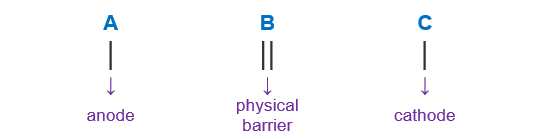# Problem: Determine the cell notation for the redox reaction given below    3 Cl2(g) + 2 Fe(s) → 6 Cl− (aq) + 2 Fe3+(aq)A) Fe(s) | Fe 3+(aq) || Cl 2(g), Cl−(aq) | PtB) Cl− (aq) | Cl 2(g) | Pt || Fe 3+(aq) | Fe(s)   C) Fe(s) | Fe3+(aq) || Cl 2(g) | Cl − (aq) | PtD) Fe(s) | Cl 2(g) || Fe 3+(aq) | Cl − (aq) | PtE) Pt | Cl 2(g) | Cl − (aq) || Fe3+(aq) | Fe(s)

###### FREE Expert Solution
95% (266 ratings)
###### FREE Expert Solution

We’re being asked to determine the cell notation for the redox reaction shown below:

3 Cl2(g) + 2 Fe(s) → 6 Cl(aq) + 2 Fe3+(aq)

When writing a cell notation, we use the following format – “as easy as ABCTo determine the cell notation of the reaction, we will use the following steps:

Step 1: Separate the reaction into two half-reactions.
Step 2: Determine the anode and the cathode.
Step 3: Write the cell notation of the reaction.

95% (266 ratings)###### Problem Details

Determine the cell notation for the redox reaction given below

3 Cl2(g) + 2 Fe(s) → 6 Cl (aq) + 2 Fe3+(aq)

A) Fe(s) | Fe 3+(aq) || Cl 2(g), Cl(aq) | Pt

B) Cl (aq) | Cl 2(g) | Pt || Fe 3+(aq) | Fe(s)

C) Fe(s) | Fe3+(aq) || Cl 2(g) | Cl  (aq) | Pt

D) Fe(s) | Cl 2(g) || Fe 3+(aq) | Cl  (aq) | Pt

E) Pt | Cl 2(g) | Cl  (aq) || Fe3+(aq) | Fe(s)

What scientific concept do you need to know in order to solve this problem?

Our tutors have indicated that to solve this problem you will need to apply the Cell Notation concept. If you need more Cell Notation practice, you can also practice Cell Notation practice problems.

What is the difficulty of this problem?

Our tutors rated the difficulty ofDetermine the cell notation for the redox reaction given bel...as high difficulty.

How long does this problem take to solve?

Our expert Chemistry tutor, Dasha took 4 minutes and 16 seconds to solve this problem. You can follow their steps in the video explanation above.

What professor is this problem relevant for?

Based on our data, we think this problem is relevant for Professor Kornienko's class at RUTGERS.